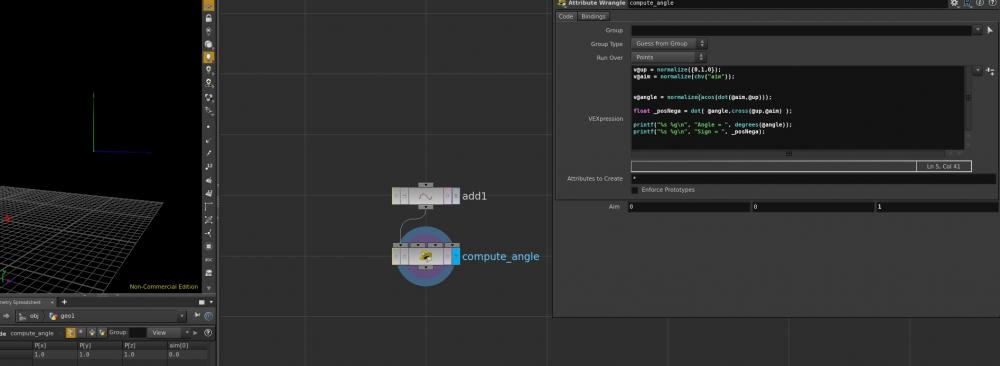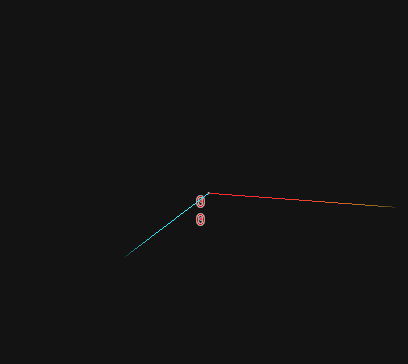Jump to content

## Recommended Posts

Hi

So I have two vectors, one is the y vector (0,1,0) and the other is an arbitrary vector called @aim, given those two I want to find the angle between those two vectors.

Now this should be pretty straight forward, just use

`acos(dot(@aim,@up))`

wich gives the angle in radiants, and converted to degrees would be a value from 0 to 180, but what If I want to find when the angle is positive and when its negative (not just the difference)?

For this I found this thread on the sidefx forums: https://www.sidefx.com/forum/topic/41091/?page=1#post-184272

And this Is what I have so far (look at the screenshot).

The "problem" here is that if I set the arbitrary vector to be (0,0,-1) for example, I get a negative 90 degree angle, so I guess there is a specific direction wich the last piece of code "looks for"...

This all comes down to know when the angle is greater than 180, but the original angle formula, as already said goes from 0-180.

There is something wrong with my code or the bheavior is normal?

If so how can I get the angle ranging from 0 to 360 degress? (I have to know when its greater than 180)

Thanks#### Share this post

##### Share on other sites

Hey,

if you want to do this on a flat plane (2d rotation) you can use atan2 instead.

otherwise you probably want to have an up vector to compare your rotation against.

then you can take the cross products between the 2 vectors.
and after that you take the dot product between that cross product and your second vector.

then see if that checking dot is positive or negative, will tell you if the rotation was clock, or counter clockwise, compared to the up vector you used.

once you know that you should be able to convert your 0-180 to a 0-360 angle.

I hope that helps#### Share this post

##### Share on other sites

So to get the angle and the positive/negative number I have to write:

```float _angle = acos(dot(@aim,@up));

float _sign = dot(@up, cross(@aim,@up));```

Because this gives me 0 as the sign variable...where is the mistake?

#### Share this post

##### Share on other sites

Second constraint must be a constant vector like @up. They define static reference frame for the changing @aim vector.

```// Point wrangle.

#define PI 3.1415926535897932384

float angle = acos(dot(v@up, v@aim));
int first_half = sign(dot(v@z, cross(v@up, v@aim))) >= 0;
angle = first_half ? angle : 2*PI - angle;

@angle = degrees(angle);```circle_angle.hipnc

•9

## Create an account or sign in to comment

You need to be a member in order to leave a comment

## Create an account

Sign up for a new account in our community. It's easy!

Register a new account

## Sign in

Already have an account? Sign in here.

Sign In Now

×
• Donations

• Leaderboard